Courses

# Relations for Enthalpy, Entropy and Internal Energy Civil Engineering (CE) Notes | EduRev

## Civil Engineering (CE) : Relations for Enthalpy, Entropy and Internal Energy Civil Engineering (CE) Notes | EduRev

The document Relations for Enthalpy, Entropy and Internal Energy Civil Engineering (CE) Notes | EduRev is a part of the Civil Engineering (CE) Course Thermodynamics.
All you need of Civil Engineering (CE) at this link: Civil Engineering (CE)

Relations for Enthalpy, Entropy and Internal Energy

One may conveniently employ the general energy relations and Maxwell equations to obtain expressions for change in enthalpy and entropy and internal energy for any process, which in turn may be used for computing the associated heat and work interactions.

Let H = H (T, P)

Then: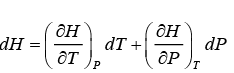But: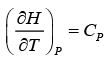Thus: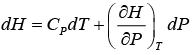...(5.16)

Using: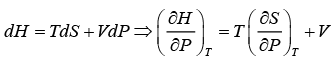...(5.17)

From Maxwell relations as in eqn. 5.15: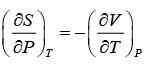...(5.18)

Thus using eqns. 5.17 and 5.18 in 5.16 we get: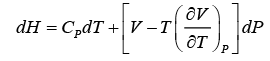...(5.20)

In the same manner starting from the general function: U = U (T,V ) andS = S (T, P ) and applying appropriate Maxwell relations one may derive the following general expressions for differential changes in internal energy and entropy.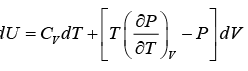...(5.21)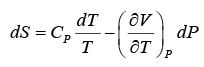...(5.22)

Or, alternately: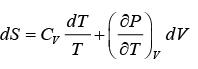...(5.23)

Thus, eqns. 5.20 to 5.23 provide convenient general relations for computing enthalpy, internal energy and entropy changes as function of volumetric properties and specific heats. If a fluid is described by a suitable EOS, these equations may be conveniently integrated to obtain analytical expressions for energy and entropy changes.

Offer running on EduRev: Apply code STAYHOME200 to get INR 200 off on our premium plan EduRev Infinity!

## Thermodynamics

56 videos|92 docs|33 tests

,

,

,

,

,

,

,

,

,

,

,

,

,

,

,

,

,

,

,

,

,

,

,

,

;